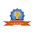# vs.eyeandcontacts.com

## Patterns, Symmetries and Basic Algebra Terms

Arithmetic progression: A sequence (series) of numbers in which there is a common difference between any two adjacent elements of the sequence. Example: 2, 4, 6, 8…

Consecutive: Numbers that follow each other in an unbroken sequence.

Half turn: A rotation of 180º about a point.

Line of symmetry: A line that divides a figure into two halves that are mirror images of each other.

Line symmetry: If a figure can be folded along a line so that the two halves match exactly, then the figure has line symmetry.

Point (central) symmetry: A figure that can be turned exactly 180º about a point and fit exactly onto itself has point symmetry.

Quarter turn: A rotation of 90º about a point.

Reflection (flip): A transformation creating a mirror image of a figure on the opposite side of a line.

Repeating decimal: A decimal which has repeating digits or a repeating pattern of digits. Example: 1/3 = 0.3333....

Revolution: A rotation of 360º about a point.

Rotation (turn): A transformation in which a figure is turned a given angle and direction around a point.

Sequence (Series): A set of numbers arranged in a special order or pattern.

Symmetry: An object is symmetrical when one half is a mirror image of the other half.

Transformation: A rule for moving every point in a figure to a new location.

Translation: A transformation that slides a figure a given distance in a given direction.

Turn centre: The point around which a figure is rotated.

Algebra: An area of mathematics where numbers are represented by letters.

Equation: A mathematical sentence that contains an = sign to show that two expressions are equal.

Expression: A combination of signs, variables, numbers and symbols that represents a mathematical relationship. Example: 3x + 2y

Inequality: A mathematical sentence that compares two unequal expressions using one of these symbols: <, >, ≤, ≥ and ≠

Variable: A letter used to represent an unknown number. Anything that does not have a fixed value.

1.1.